Courses

# NEET Previous Year Questions (2014-20): Chemical Bonding and Molecular Structure Notes | EduRev

## Class 11 : NEET Previous Year Questions (2014-20): Chemical Bonding and Molecular Structure Notes | EduRev

The document NEET Previous Year Questions (2014-20): Chemical Bonding and Molecular Structure Notes | EduRev is a part of the Class 11 Course Chemistry Class 11.
All you need of Class 11 at this link: Class 11

Q.1. Identify a molecule which does not exist.     (2020)
(a) C2
(b) O2
(c) He2
(d) Li2
Ans: c
Diatomic molecule for which bond order is zero or negative does not exist.

 Molecule BO C2 2 O2 2 He2 Zero Li2 1

Q.2. The calculated spin only magnetic moment of Cr2+ ion is :      (2020)
(a) 5.92 BM
(b) 2.84 BM
(c) 3.87 BM
(d) 4.90 BM
Ans: d
Cr2+ → 1s2 2s2 2p6 3s2 3p6 3d4
∴ n (number of unpaired electrons) = 4
∴ μ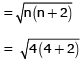= 4.8989
= 4.9 B.M.

Q.3. Which of the following set of molecules will have zero dipole moment ?      (2020)
(a) Nitrogen trifluoride, beryllium difluoride, water, 1,3-dichlorobenzene
(b) Boron trifluoride, beryllium difluoride, carbon dioxide, 1,4-dichlorobenzene
(c) Ammonia, beryllium difluoride, water 1,4-dichlorobenzene
(d) Boron trifluoride, hydrogen fluoride, carbon dioxide, 1,3-dichlorobenzene
Ans: b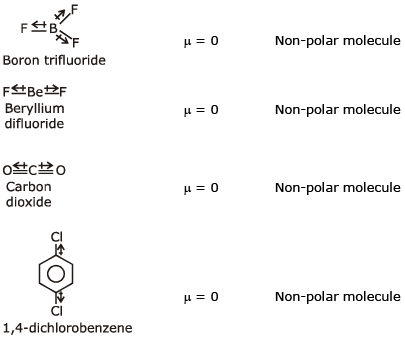Q.4. Which of the following diatomic molecular species has only π bonds according to Molecular Orbital Theory?    (2019)
(a) O2
(b) N2
(c) C2
(d) Be2
Ans:
c
MO configuration C2 is: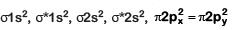Q.5. Identify the incorrect statement related to PCl5 from the following:    (2019)
(a) Three equatorial P–Cl bonds make an angle of 120° with each other
(b) Two axial P–Cl bonds make an angle of 180° with each other
(c) Axial P–Cl bonds are longer than equatorial P–Cl bonds
(d) PCl5 molecule is non-reactive
Ans:
d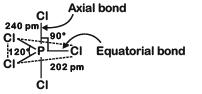(1) True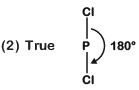(3) True
Axial bond : 240 pm
Equatorial bond : 202 pm
(4) False
Due to longer and hence weaker axial bonds, PCl5 is a reactive molecule.

Q.6. Consider the following species:
CN+, CN, NO and CN
Which one of these will have the highest bond order?    (2018)
(a) NO
(b) CN
(c) CN+
(d) CN
Ans:
b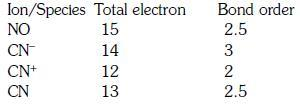Q.7. Which of the following molecules represents the order of hybridisation sp2, sp2, sp, sp from left toright atoms ?    (2018)
(a) HC ≡ C – C ≡ CH
(b) CH2 = CH – C ≡ CH
(c) CH2 = CH – CH = CH2
(d) CH3 – CH = CH – CH3
Ans:
b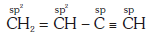Q.8. Which of the following pairs of compounds is isoelectronic and isostructural ?    (2017)
(a)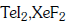(b)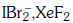(c)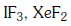(d)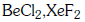Ans:
b
IBr2- ,XeF2
Total number of valence electrons are equal in both the species and both the species exhibit linear shape.

Q.9. Which of the following pairs of species have the same bond order ?    (2017)
(a) O2, NO+
(b) CN, CO
(c) N2 , O2-
(d) CO, NO
Ans:
b
Total no. of electrons in CN is 14
Total no. of electrons in CO is also 14
Hence, B.O. of both CN & CO is 3

Q.10. Predict the correct order among the following :    (2016)
(a) Ione pair - bond pair > bond pair - bond pair > lone pair - lone pair
(b) lone pair - lone pair > lone pair - bond pair > bond pair - bond pair
(c) lone pair - lone pair > bond pair - bond pair > lone pair - bond pair
(d) bond pair - bond pair > lone pair - bond pair > lone pair - lone pair
Ans:
b
The order of repulsion force according to VSEPR theory :
lone pair - lone pair > lone pair - bond pair > bond pair - bond pair

Q.11. Consider the molecules CH4, NH3 and H2O. Which of the given statement is false ?    (2016)
(a) The H-C-H bond angle in CH4 is larger than the H-N-H bond angle in NH3
(b) The H-C-H bond angle in CH4, the H-N-H bond angle in H2O are all greater than 90°.
(c) The H-O-H bond angle in H2O  is larger than the H-C-H bond angle in CH4.
(d) The H-O-H bond angle in H2O is smaller than the H-N-H bond angle in NH3.
Ans:
c
The H-O-H bond angle in H2O  is larger than the H-C-H bond angle in CH4.
According to VBT: lone pair-lone pair repulsion is more than a bond pair -lone pair repulsion.
As the number of lone pair of electrons on central element increases, repulsion between that lone pair of electrons increases and therefore, bond angle decreases.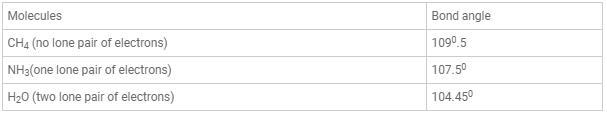Q.12. The correct bond order in the following species is:    (2015)
(a)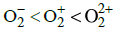(b)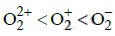(c)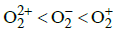(d)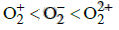Ans:
a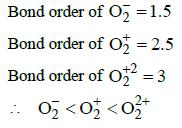Q.13. Which one of the following species has plane triangular shape ?    (2014)
(a)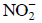(b) CO2
(c) N3
(d)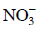Ans:
d
Species with sp2 hybridisation are plane triangular in shape. Among the given species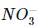is sp2 hybridised with no lone pair of electrons on central atom, N. Whereas, N3,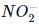and CO2 are sp hybridised with a linear shape.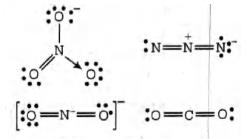Q.14. Which of the following molecules has the maximum dipole moment ?    (2014)
(a) NH3
(b) NF3
(c) CO2
(d) CH
4
Ans: a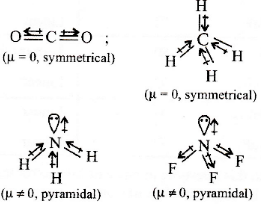In NH3, H is less electronegative than N and hence dipoie moment of each N - H bond is towards N and create high net dipole moment whereas in NF3 ,F is more electronegative than N, the dipole moment of each N - F bond is opposite to that of lone pair, hence reducing the net dipole moment.

Q.15. Be2+ is isoelectronic with which of the following ions ?    (2014)
(a) Na+
(b) Mg2+
(c) H+
(d) Li+
Ans:
a
Isoelectronic species contain same number of electrons.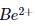contains 2 electrons.
Among the given options, only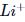contains 2 electrons and therefore, it is isoelectronic with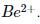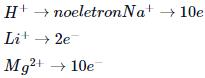Hence,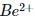is isoelectronic with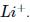Offer running on EduRev: Apply code STAYHOME200 to get INR 200 off on our premium plan EduRev Infinity!

## Chemistry Class 11

129 videos|213 docs|188 tests

,

,

,

,

,

,

,

,

,

,

,

,

,

,

,

,

,

,

,

,

,

;